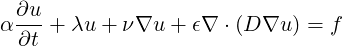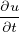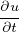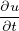### 7.1 Synopsis

The ADRSolver is designed to solve partial differential equations of the form:(7.1)

in either discontinuous or continuous projections of the solution field. For a full list of the equations which are supported, and the capabilities of each equation, see the table below.

 Equation to solve EquationType Dimensions Projections u = f `Projection` All Continuous/Discontinuous ∇2u = 0 `Laplace` All Continuous/Discontinuous ∇2u = f `Poisson` All Continuous/Discontinuous ∇2u + λu = f `Helmholtz` All Continuous/Discontinuous ϵ∇2u + V∇u = f `SteadyAdvectionDiffusion` 2D only Continuous/Discontinuous ϵ∇2u + λu = f `SteadyDiffusionReaction` 2D only Continuous/Discontinuous ϵ∇2u + V∇u + λu = f `SteadyAdvectionDiffusionReaction` 2D only Continuous/Discontinuous+ V∇u = f `UnsteadyAdvection` All Continuous/Discontinuous= ϵ∇2u `UnsteadyDiffusion` All Continuous/Discontinuous= ϵ∇2u + R(u) `UnsteadyReactionDiffusion` All Continuous+ V∇u = ϵ∇2u `UnsteadyAdvectionDiffusion` All Continuous/Discontinuous+ u∇u = 0 `UnsteadyInviscidBurger` 1D only Continuous/Discontinuous

Table 7.1: Equations supported by the ADRSolver with their capabilities.